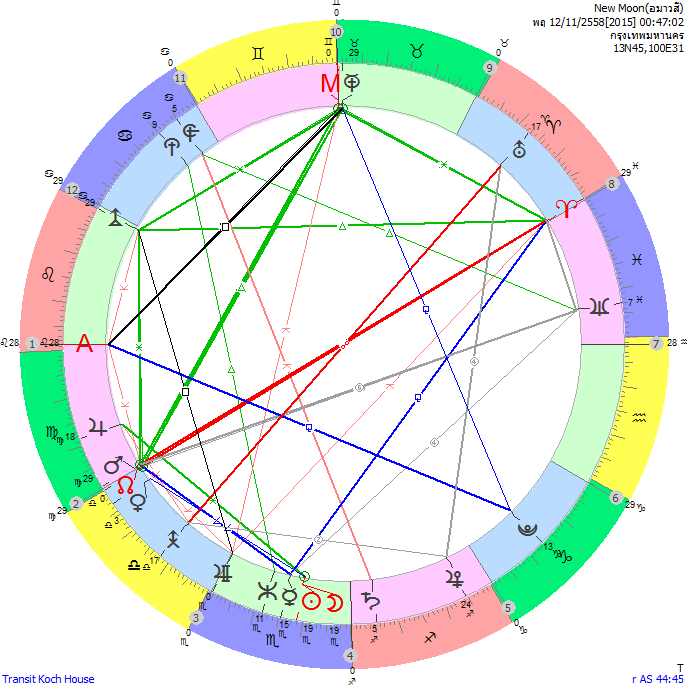New Moon 12/11/2558 00:47 โพสต์ 10 พ.ย.58 09:59 น. : อ่าน 6535AR
= NO = MA = ME[-135] = NE[-22.5]
= AS/AP = NO/VE = NO/MA = VE/MA = CU/HA = CU/KR
= MC/VU = AD/VU
= MC/AP = AS/VU = JU/KR = AP/AD = SU/PO[-135] = MO/PO[-135] = JU/PL[-135]
= UR/VU[67.5]
= SU/CU[-112.5] = MO/CU[-112.5] = PL/AP[-112.5]
= AS/JU[157.5] = HA/PO[157.5] = NO/ME[-157.5] = ME/MA[-157.5] = VE/PO[-157.5] = PL/VU[-157.5]

SU, MO
= VE[-45]
= CU/ZE = SA/AP
= AR/HA = AR/KR = NE/VU
= NO/HA[-90] = NO/KR[-90] = VE/HA[-90] = MA/KR[-90] = AD/PO[-90]
= NE/AP = PL/CU = JU/ZE[-45]
= AS/CU[-22.5] = JU/SA[-22.5]
= ME/HA[-67.5] = KR/PO[-67.5]
= MC/CU[112.5] = CU/AD[112.5]

MC
= AD = AS
= AR/VU = UR/KR
= PL/ZE = CU/AP
= NO/VU = VE/VU = MA/VU = ZE/KR = UR/PL[-90]
= NO/AP = MA/AP = JU/PO = AR/AP[-135]
= AS/UR[22.5] = UR/AD[-22.5] = NE/KR[-22.5]
= AS/ZE[112.5] = ME/VU[112.5] = SA/KR[112.5]
= ME/AP[157.5] = JU/CU[157.5]

AS
= MC[-90] = AD[-90] = PL
= NO/VU = MA/VU = ZE/KR = HA/ZE
= UR/PL
= SU/SA = MO/SA = PL/ZE = CU/AP = AR/VU[-90] = MC/AD[-90] = UR/KR[-90]
= MA/AP
= SU/NE = MO/NE
= SA/HA[22.5] = VU/PO[22.5]
= JU/CU[67.5] = AP/PO[67.5]
= VE/NE[112.5] = NE/HA[-112.5]
= MC/PL[-157.5] = PL/AD[-157.5]เห-มายัน(Winter Solstice) 22/12/2558 11:48New Moon 12/11/2558 00:47New Moon 14/08/2558 21:53เห-มายัน(Winter Solstice) 22/12/2557 06:03Solar Eclipse 24/10/2557 04:57วสันตวิษุวัต(Vernal Equinox) 20/03/2557 23:57New Moon 01/03/2557Full Moon 15/02/2557 06:53New Moon 31/01/2014 04:38New Moon 01/01/2014 18:14Winter Solstice 22/12/2013 00:11New Moon 03/12/2013Full Moon 17/11/2013New Moon(Solar Eclipse)03/11/2013Full Moon(Lunar Eclipse)19/10/2013New Moon 05/10/2013ศารทวิษุวัต 23/09/2013Full Moon 19/09/2013New Moon 05/09/2013